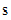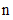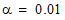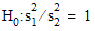# NMath User's Guide

41.6 Two Sample F-Test (.NET, C#, CSharp, VB, Visual Basic, F#)

Class tests whether the variances of two populations are equal. For example, suppose random samples from two normal populations are taken. The first sample consists of 10 observations with a standard deviation of 5.203; the second sample consists of 25 observations with a standard deviation of 2.623. At the 0.10 significance level, is there sufficient evidence to suggest that the populations from which these samples were drawn have equal variances?

As described Section 41.1, all hypothesis test classes provide two paths for constructing instances of that type: a parameter-based method and a data-based method. Thus, you can construct a TwoSampleFTest object by explicitly specifying the standard deviation (),and size () of each sample, like so:

Code Example – C# F-test

```double s1 = 5.203;
```
```int n1 = 10;

```

```double s2 = 2.623;
```
```int n2 = 25;

```

```var test = new TwoSampleFTest( s1, n1, s2, n2 );
```

Code Example – VB F-test

```Dim S1 As Double = 5.203
```
```Dim N1 As Integer = 10

```

```Dim S2 As Double = 2.623
```
```Dim N2 As Integer = 25

```

```Dim Test As New TwoSampleFTest(S1, N1, S2, N2)
```

Or by supplying two sets of sample data. For instance, if the sample data is in two vectors v1 and v2:

Code Example – C# F-test

```var test = new TwoSampleFTest( v1, v2 );
```

Code Example – VB F-test

```Dim Test As New TwoSampleFTest(V1, V2)
```

The sample standard deviations and sizes are calculated from the given data.

In addition to the properties common to all hypothesis test objects (Section 41.1), a TwoSampleFTest object provides the following read-only properties:

S1 and S2 get the standard deviations of the samples.

N1 and N2 get the sizes of the samples.

DegreesOfFreedom1 gets the numerator degrees of freedom.

DegreesOfFreedom2 gets the denomenator degrees of freedom.

By default, a TwoSampleFTest object performs a two-sided hypothesis test () with. Non-default test parameters can be specified at the time of construction using constructor overloads, or after construction using the provided Type and Alpha properties.

Once you've constructed and configured a TwoSampleFTest object, you can access the various test results using the provided properties, as described in Section 41.1:

Code Example – C# F-test

```Console.WriteLine( "t-statistic = " + test.Statistic );
```
```Console.WriteLine( "numerator df = " + test.DegreesOfFreedom1 );
```
```Console.WriteLine( "denomenator df = " + test.DegreesOfFreedom2 );
```
```Console.WriteLine( "p-value = " + test.P );
```
```Console.WriteLine( "reject the null hypothesis? " + test.Reject);
```

Code Example – VB F-test

```Console.WriteLine("t-statistic = " & Test.Statistic)
```
```Console.WriteLine("numerator df = " & Test.DegreesOfFreedom1)
```
```Console.WriteLine("denomenator df = " & Test.DegreesOfFreedom2)
```
```Console.WriteLine("p-value = " & Test.P)
```
```Console.WriteLine("reject the null hypothesis? " & Test.Reject)
```

The output is:

```F-statistic = 3.93469497446923
```
```numerator df = 9
```
```denomenator df = 24
```
```p-value = 0.00693561186501657
```
```reject the null hypothesis? True
```

This indicates that we cannot reject the null hypotheses ().

Finally, remember that the ToString() method returns a formatted string representation of the complete test results:

```Two Sample F Test
```
```-----------------

```

```Sample Sizes = 10 and 25
```
```Standard Deviations = 5.203 and 2.623
```
```Variances = 27.071209 and 6.880129
```
```Computed F statistic: 3.93469497446923, num df = 9, denom df = 24

```

```Hypothesis type: two-sided
```
```Null hypothesis: true ratio of variances = 1
```
```Alt hypothesis: true ratio of variances != 1
```
```P-value: 0.00693561186501657
```
```REJECT the null hypothesis for alpha = 0.01
```
```0.99 confidence interval: 1.06490202325594 22.5425454339445
```

Top

Top